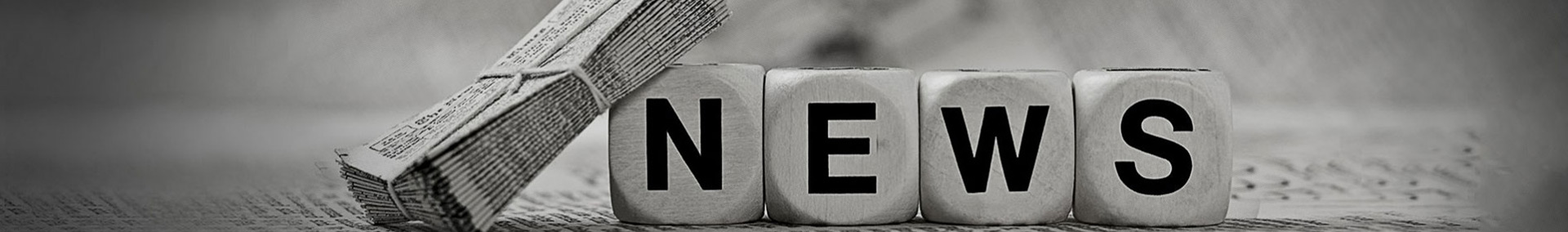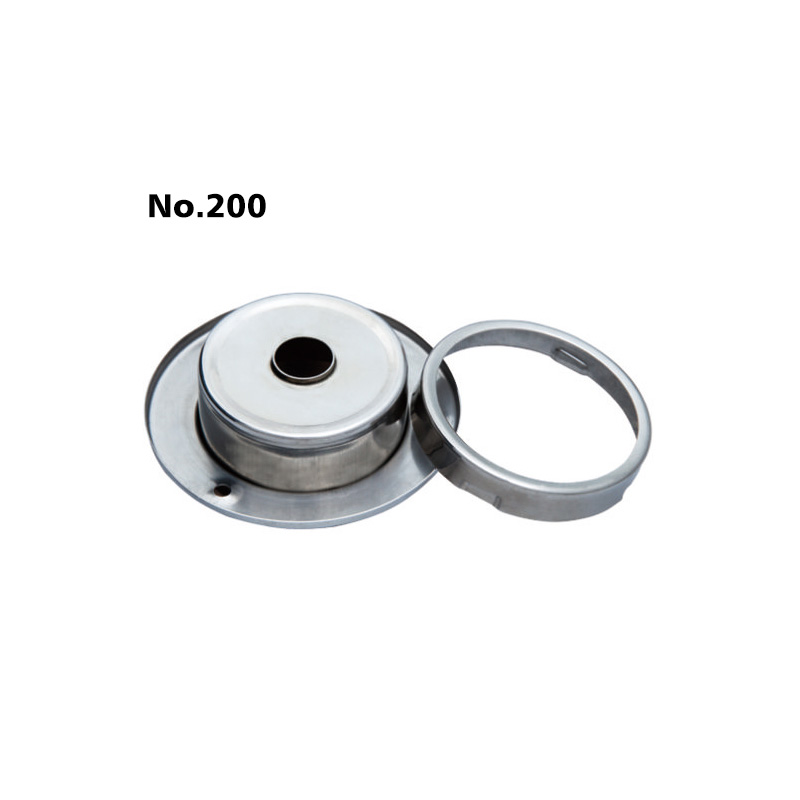# How to use the two-dimensional measuring instrument?

Update:03 Dec 2020
Summary:

The two-dimensional detection instrument is used to mea […]

The two-dimensional detection instrument is used to measure: angle, diameter, radius, distance from point to line, eccentricity of two circles, distance between two points, etc. The two-dimensional detection instrument is a two-dimensional measurement, and the principle is similar to that of a projector; but different data processing systemsElectric Contacters For Pressure Gauge have different measurement and calculation methods. The two-dimensional detection instrument generally uses CCD to take the image into the computer for measurement and calculation. Some functions may not be done, depending on whether the projector is equipped with a data processor, the surface measurement effect is not good.
1. Tangent method.

The tangent method refers to the method of manually rotating the engraved lines on the screen bai or the lens, respectively aligning the two edges of the workpiece, and measuring the angle through the encoder or circular grating counting. This method is divided into two, projection tangent method, such as projector, tool measuring microscope, etc., and image tangent method, such as imager, video microscope with video function, relying on the software's own meter line rotation measurement. The tangent method is convenient and simple to operate, but the measurement intensive reading is low, and it is suitable for rapid batch inspection.2. Point calculation method.

The point calculation method is more suitable. All geometric elements are composed of points, including the basic elements of straight lines, curves and arcs. The two-dimensional plane angle is composed of two straight lines of basic geometric elements, which are composed of countless points. Therefore, whether the angle measurement is accurate or not is the most critical point.